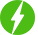Differential Equations
• Show all
• Hottest
• Popular
1

## Differential Equations

Differential Equations, First Order First Degree Differential Equation: Exact & Linear Differential Equation & Bernoulli's Differential Equation. ...

ExpandRegister New Account
• Total (0)
0